，或许大家听起来并不陌生。早在十几年前，当时不论是文字游戏“泥巴(Mud)”或是交友、社交网站，我们只能通过屏幕上的文字来传达与交互信息；随着技术不断进步，2D/3D图形技术高速崛起，通过在基础模型上由客户随意挑选、任意更换各种造型(素材)，即可打造出真正属于“自我”独特风格的网络虚拟形象，QQ秀便是我们耳熟能详的代表，更贴近真实的如(RPG)游戏及虚拟现实中的换装/换肤系统同样亦得益于纸娃娃机制。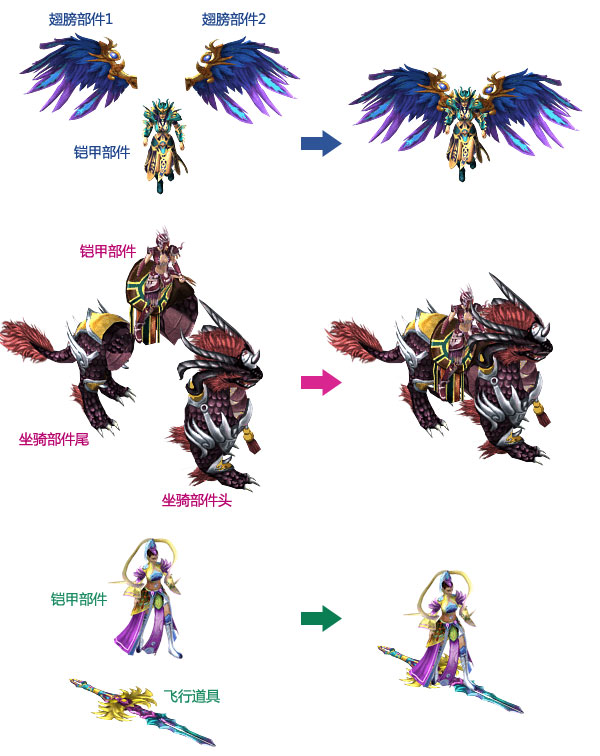2D/2.5D游戏中角色带翅膀飞行要考虑左右翼与身体的层次关系，骑马则需要考虑马头/马尾与身体间的层次问题。而且武器长短，角色朝向，行为姿势等也都可能影响到各部件的层次关系。因此，一些游戏为了简化设计，同时又不失华丽，便诞生了比如“踏云”，“御剑”，“乘鹤”，“踩蝶”等诸多天马行空的驾驭模式，这些乘具的共同点就是均被踩在脚上，自然而然处理起来更简单明了。当然，如果角色是3D模型的话则无需考虑这么多层叠关系。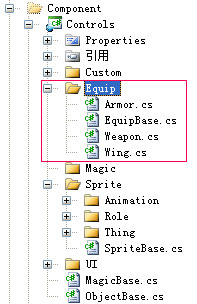/// <summary>

/// 装备部件基类

/// </summary>
public abstract class EquipBase : ObjectBase {

/// <summary>

/// 加载完毕

/// </summary>

/// <summary>

/// 获取或设置部件名

/// </summary>
protected string partName { getset; }

long index = 0//异步加载与换装同步协调
public override int Code {

get { return base.Code; }

set {
index
++;

if (value == -1) { base.Code = value; return; }

string key = string.Format("{0}{1}", partName, value);

if (Res.ContainsKey(key)) {

base.Code = value;
}
else {
string.Format("{0}{1}.xap", partName, value), string.Format("{0},{1}", index, value), 2000);
}
}
}

string[] str = e.UserState.ToString().Split(',');

if (Convert.ToInt64(str) == index) {

int code = Convert.ToInt32(str);

string key = string.Format("{0}{1}", partName, str);

if (!Res.ContainsKey(key)) { Res.Add(key, new StreamResourceInfo(e.Result as Stream, "application/binary")); }

base.Code = code;
}
}

Dictionary
<string, Point> frameOffset = new Dictionary<string, Point>(); //各帧偏移
/// <summary>

/// 加载配置

/// </summary>
XElement info
FullName
= info.Attribute("FullName").Value;

//解析各帧偏移
IEnumerable<XElement> iFrame = info.Element("Frames").Elements();
frameOffset.Clear();

foreach (XElement element in iFrame) {
"ID").Value, new Point() {
X
= (double)element.Attribute("OffsetX"),
Y
= (double)element.Attribute("OffsetY"),
});
}

}

bool _IsTurn;

/// <summary>

/// 获取或设置是否水平翻转

/// </summary>
public bool IsTurn {

get { return _IsTurn; }

set {

if (_IsTurn != value) {
Transform
= (_IsTurn = value) ? scaleTransform : null;
}
}
}

bool _Flash;

/// <summary>

/// 获取或设置是否闪光

/// </summary>
public bool Flash {

get { return _Flash; }

set {

if (_Flash != value) {

//if (_Flash = value) {

//    dispatcherTimer.Start();

//} else {

//    this.Opacity = 1;

//    dispatcherTimer.Stop();

//}
this.Opacity = (_Flash = value) ? 0.4 : 1;
}
}
}

bool order = false;
DispatcherTimer dispatcherTimer
= new DispatcherTimer() { Interval = TimeSpan.FromMilliseconds(100) }; //换装时的闪光特效计时器
public EquipBase() {
dispatcherTimer.Tick
+= new EventHandler(dispatcherTimer_Tick);
}

void dispatcherTimer_Tick(object sender, EventArgs e) {

if (order) {

this.Opacity = this.Opacity + 0.1;

if (this.Opacity >= 1) { order = false; }
}
else {

this.Opacity = this.Opacity - 0.1;

if (this.Opacity <= 0.3) { order = true; }
}
}

static Dictionary<string, Stream> equipRes = new Dictionary<string, Stream>();

ScaleTransform scaleTransform
= new ScaleTransform() { ScaleX = -1 };

/// <summary>

/// 呈现帧图

/// </summary>
public void Display(string key) {

string resKey = string.Format("{0}{1}{2}", partName, Code, key);

if (!equipRes.ContainsKey(resKey)) {
string.Format("{0}{1}", partName, Code)], new Uri(string.Format("{0}.png", key), UriKind.Relative)).Stream);
}

this.StreamSource = equipRes[resKey];

this.InternalOffset = frameOffset[key];

if (IsTurn) { scaleTransform.CenterX = Center.X - frameOffset[key].X; }
}

public override void Dispose(object sender, EventArgs e) {
dispatcherTimer.Stop();
dispatcherTimer.Tick
-= dispatcherTimer_Tick;

base.Dispose(sender, e);
}
}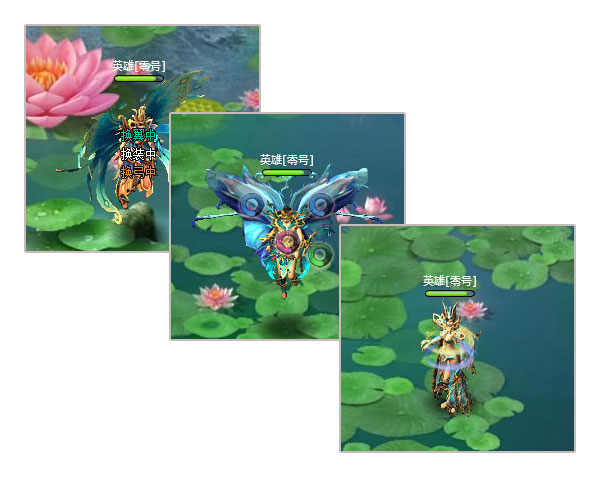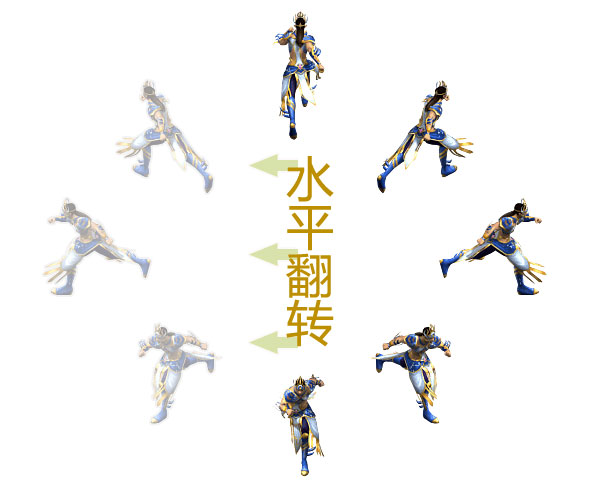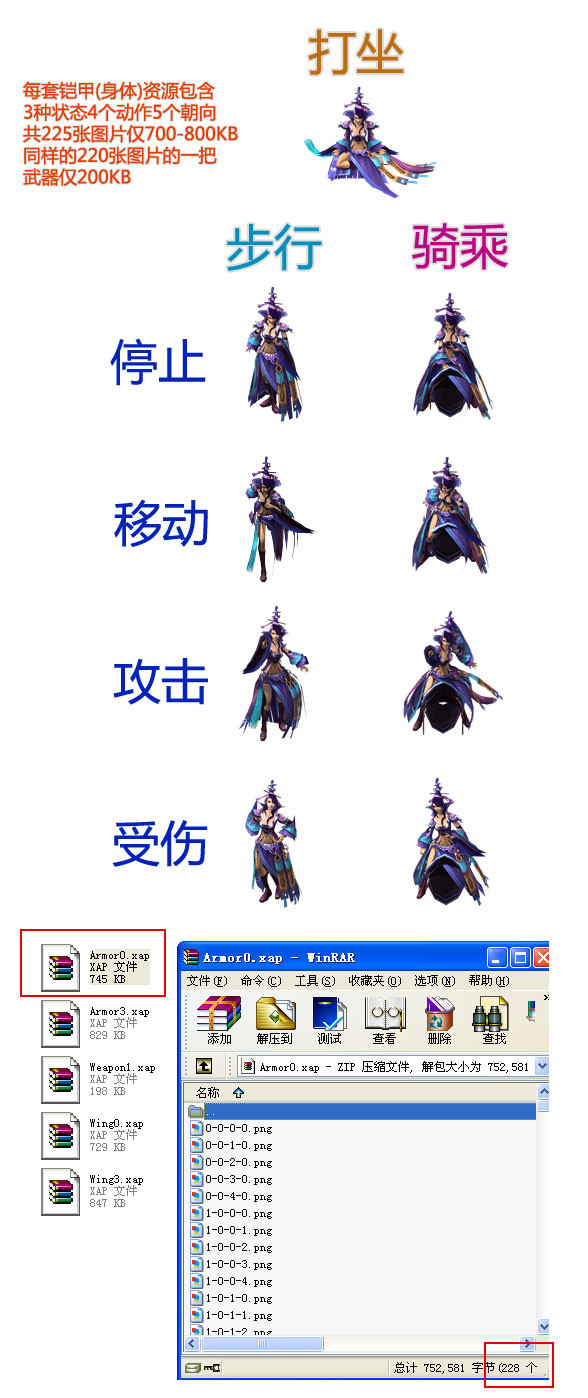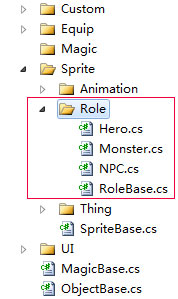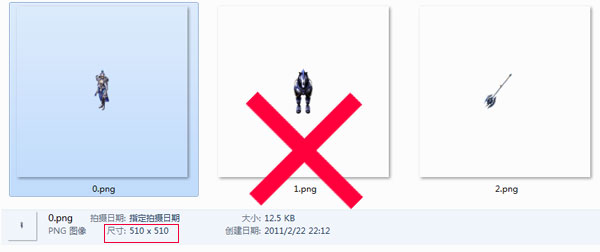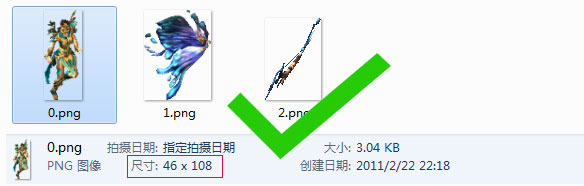posted on 2011-02-22 23:07  深蓝色右手  阅读(14511)  评论(28编辑  收藏  举报